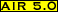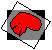# reslice_vector## Purpose:

This program takes a vector field file and uses the information that it contains to load the corresponding image file and generate a new, realigned file.

## Usage:

reslice_vector vector-field output [options]

options:
[-a alternate-reslice-file]
changes the file to be resliced
[-d divide-by-intensity-scale-factor]
scales output by dividing by specified factor
[-df divide-by-intensity-scale-factor-file]
scales output by dividing by factor specified in file
[-n model {half-window-widths}]
changes interpolation model
[-o]
grants permission to overwrite output
[-s multiplicative-intensity-scale-factor]
scales output using specified multiplicative factor
[-sf multiplicative-intensity-scale-factor-file]
scales output using multiplicative factor specified in file
[-w .warp-file]
a .warp file to be combined with the vector field
where the following definitions apply:
alternate-reslice-file [-a]
name of an alternate reslice file that is spatially equivalent (same voxel sizes and dimensions) to the default reslice file specified in the vector field file
if the -w option is used to define a .warp file, the alternate reslice file must be spatially equivalent to the default reslice file specified in the .warp file
divide-by-intensity-scale-factor [-d]
output intensities are divided by this positive value
divide-by-intensity-scale-factor-file [-df]
output intensities are divided by the value in this ASCII file containing a single positive number
half-window-width [-n ...]
half the width of the window (in whole voxels) to apply when doing sinc interpolation
larger values are more accurate, but considerably slower
model [-n ...]
the interpolation model number, selected from the menu:
• 0. nearest neighbor
• 1. trilinear
• 2. windowed sinc in original xy plane, linear along z
• 3. windowed sinc in original xz plane. linear along y
• 4. windowed sinc in original yz plane, linear along x
• 5. 3D windowed sinc
multiplicative-intensity-scale-factor [-s]
output intensities are multiplied by this positive value
multiplicative-intensity-scale-factor-file [-sf]
output intensities are multiplied by the value in this ASCII file containing a single positive number
output [mandatory]
name of the output image file (.hdr or .img suffix optional)
vector-field [mandatory]
name of the vector-field file
.warp-file [-w]
name of the .warp-file that will be combined with the vector-field. The standard file of the .warp file must match the reslice file of the vector-field file. The file that is loaded and resliced will be the reslice file identified in the .warp file

## Examples:

reslice_vector mri.vectorfield newmri -o
• The reslice file identified in mri.vectorfield will be resampled based on the nonlinear spatial transformation parameters in mri.vectorfield. The newly created file will be called newmri.img and will overwrite any existing file with that name.

• Unlike reslice, this program does not interpolate output to create cubic voxels.
• Trilinear interpolation is the default interpolation method for this program.
• The half window widths for sinc interpolation control the number of surrounding voxels that contribute to the interpolated value along each axis of the reslice file. A half-window width of 1 results in interpolation along that axis that only includes the nearest neighbor on either side and a half-window width of 6 will include the six nearest neighboring voxels in either direction. The total number of voxel included for standard 3D sinc interpolation is therefore 8*(x_half_window_width)*(y_half_window_width*(z_half_window_width). The larger the half windows,the more closely the interpolation will match true sinc interpolation, but also the slower the resampling process.
• Windowing of sinc interpolation is implemented using a Hanning window function exactly as described by Hajnal JV, Saeed N, Soar EJ, Oatridge A, Young IR, Bydder GM. Journal of Computer Assisted Tomography 1995:19:289-296.

## Error messages: (alphabetical by case)

-a must be followed by an image file name
• supply an image file name; this file must have the same dimensions and voxel sizes as the reslice file specified in the vector field file (or in the .warp file if the -w option is used). The programs scan_vector and scanheader will allow you to verify this.
-d must be followed by a positive number
• supply a positive number
-df must be followed by a file name
• supply a file name; this must be a plain text ASCII file containing a single number
-n ____ does not define a valid interpolation model
• choose a model number from the menu
-n ____ must be followed by three positive integers
• certain sinc interpolation models require three half-window-widths
-n ____ must be followed by two positive integers
• certain sinc interpolation models require two half-window-widths
-n must be followed by a valid interpolation model number
• choose a model number from the menu
-s must be followed by a positive number
• supply a positive number
-sf must be followed by a file name
• supply a file name; this must be plain text ASCII file containing a single number
-w must be followed by a .warp file name
• supply the name of a .warp file. The standard file in this .warp file must match the reslice file in the vector field file
Unable to parse ____
• check syntax for undefined flags
Unable to parse argument ____, which was expected to begin with a '-'
• check syntax, an argument without a flag is positioned as if were a flagModified: December 15, 2001